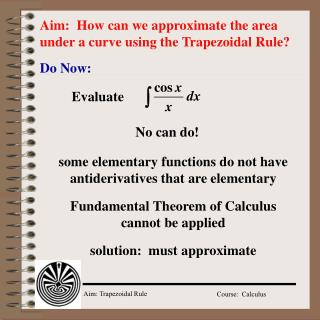Download PresentationAim: How can we approximate the area under a curve using the Trapezoidal Rule?Aim: How can we approximate the area under a curve using the Trapezoidal Rule? - PowerPoint PPT Presentation

Download PresentationAim: How can we approximate the area under a curve using the Trapezoidal Rule?
An Image/Link below is provided (as is) to download presentation

Download Policy: Content on the Website is provided to you AS IS for your information and personal use and may not be sold / licensed / shared on other websites without getting consent from its author. While downloading, if for some reason you are not able to download a presentation, the publisher may have deleted the file from their server.

- - - - - - - - - - - - - - - - - - - - - - - - - - - E N D - - - - - - - - - - - - - - - - - - - - - - - - - - -
Presentation Transcript

1. Aim: How can we approximate the area under a curve using the Trapezoidal Rule? Do Now: Evaluate No can do! some elementary functions do not have antiderivatives that are elementary Fundamental Theorem of Calculus cannot be applied solution: must approximate

2. a b x1 x2 x3 x4 The Trapezoidal Rule x0 x5 partition into equal subintervals

3. f(x0) x1 f(x1) x0 The Trapezoidal Rule Area of 1st Trapezoid Area of ith Trapezoid Total Area is sum of all Trapezoids

4. a b x1 x2 x3 x4 Total Area is sum of all Trapezoids The Trapezoidal Rule 1 2 3 4 5 x0 x5

5. a b x1 x2 x3 x4 The Trapezoidal Rule 1 2 3 4 5 x0 x5

6. Trapezoidal Rule Let f be continuous on [a, b]. The Trapezoidal Rule for approximating is given by

7. Model Problem Use the Trapezoidal Rule to approximate and compare results for n = 4 and n = 8 0 0

8. Model Problem n = 4 n = 8

9. left endpoint right endpoint midpoint Approximation Rules

10. Trapezoidal Rule Approximation Rules

11. Model Problem 16.328125

12. Model Problem